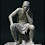## 20110429

### 1 = 0

x = y

x2 - y2 = xy - y2

(x + y)(x - y) = (x - y)y

x + y = y

2y = y

2 = 1

1 = 0

#### 21 則留言:

1.Because it's assumed x=y in the beginning.
(p.s. i learnt this from Alex before)

2.catcat,

Who's Alex? And why is assuming x=y a problem?

3.因為 x=y > x-y=0
所以唔可以做分母呢 :)

4./*
兩邊都除以 (x - y)，便得出：

x + y = y
*/

since x=y, dividing by (x-y) is the same as dividing by 0. therefore, you cannot step forward to x + y = y

5.兩邊都除以 (x - y)，便得出：
x + y = y

====================================

x=y
x-y=0

So you cannot divide it directly?

6.Because one cannot divide both sides of a equation with 0. As it is possible that (x-y) and y is zero, they cannot be divided from both sides of equation.

7.well sorry, x-y *is* zero in this equation.

8.hehe :)

9.我估應該要考究一下點解任何數都唔可以除"0"
任何數除"0"為何定義為indefinite？

如果任何數除"0"係definite，咁樣即是 0/0 = 1
咁會好大件事...
e.g.
0 * (x + y - 123) = 0 * (x - y -456)
唔知自己計緊乜，哈哈...

總之若果 x = y，計到要除(x - y)的時候已經產生邏輯不合法的情況，因為(x - y)一定會係"0"

至於問題本身，其實可以著重於任何數除"0"為何定義為indefinite？

10.x + y = y
=>
x = 0

11.Another challenge:

Sqrt((-1)^2) = Sqrt(1) = 1

Let sqrt(-1) = i, so i^2 = -1
Sqrt((-1)^2) = (Sqrt(-1))^2 = i^2 = -1

1 = -1

12.Division by zero !

13.看來這對大家來說太容易了，我不一一回應喇！

14.Matt:
There are two types of information in an complex number: magnitude and direction.
When you square an complex number, you can calculate the area of a square in which the length of each side is equivalent to magnitude of the complex. And no matter how you rotate the square, its area would be the same. That's mean that there is loss in information (that of direction) in the process.
The notion 1=-1 is not "untrue", but it is "not the whole story of the truth" by eliminating the information on direction.

15.Charles Mak's brother4/30/2011 8:14 上午

2y = y is not = 2 = 1

16.Charles Mak's brother4/30/2011 8:23 上午

因我地做數嗰陣唔會調個未知數過去

17.Charles Mak's brother,

//2y = y is not = 2 = 1//

2y = y
(2y)/y = y/y
2=1

18.Charles Mak's brother4/30/2011 9:08 上午

x2 - y2 = xy - y2 is = 0

19.Charles Mak's brother,

Yes, 0 = 0, not a problem!

20.y is 0.
if we divide y on each side then we get 2=1
but this is inconsistent with the real numbers and it is a contracdiction to the fact.
thus we conclude that x=y=0.
I think we call this prof by contracdiction in mathematics. we use this technique to show the hypithesis we made is wrong.

21.Mansfield,

I thought you knew that this is not a real proof!